| Next || Previous || Top |

## EXCHANGE_SPECIES

This keyword data block is used to define a half-reaction and relative log K for each exchange species. Normally, this data block is included in the database file and only additions and modifications are included in the input file.

###### Example data block
`Line 0:  EXCHANGE_SPECIES `
`Line 1a:	X- = X-`
`Line 2a:		log_k		0.0`
`Line 1b:	X- + Na+ = NaX`
`Line 2b:		log_k		0.0`
`Line 3: 		-gamma		4.	0.075	0.1`
`Line 1c: 	2X- + Ca+2 = CaX2`
`Line 2c:		log_k		0.8`
`Line 4:		-davies`
`Line 1d:	Xa- = Xa-`
`Line 2d:		log_k		0.0`
`Line 1e:	Xa- + Na+ = NaXa`
`Line 2e:		log_k		0.0`
`Line 1f:	2Xa- + Ca+2 = CaXa2`
`Line 2f:		log_k		2.0`
###### Explanation

Line 0: EXCHANGE_SPECIES

Keyword for the data block. No other data are input on the keyword line.

Line 1: Association reaction

Association reaction for exchange species. The defined species must be the first species to the right of the equal sign. The association reaction must precede any identifiers related to the exchange species. Master species have an identity reaction (Lines 1a and 1d).

Line 2: log_k log K

log_k --Identifier for log K at 25 °C. Optionally, -log_k , logk , -l [ og_k ], or -l [ ogk ].

log K --Log K at 25 °C for the reaction. Unlike log K for aqueous species, the log K for exchange species is implicitly relative to a reference exchange species. In the default database file, sodium (NaX) is used as the reference and the reaction X - + Na + = NaX is given a log K of 0.0 (Line 2b). By subtracting the reaction for NaX in Line 1b twice from the reaction for CaX2 in Line 1c, it follows that log K for the reaction in Line 2c is numerically equal to log K for the reaction 2NaX + Ca +2 = CaX 2 + 2Na + . The identity reaction for a master species has log K of 0.0 (Lines 2a and 2d); reactions for reference species also have log K of 0.0 (Lines 2b and 2e). Default is 0.0.

Line 3: -gamma Debye-Hückel a, Debye-Hückel b, active_fraction_coefficient

-gamma --Indicates WATEQ Debye-Hückel equation will be used to calculate an activity coefficient for the exchange species if the aqueous model is an ion-association model (see -exchange_gammas in the EXCHANGE data block for information about activity coefficients when using the Pitzer or SIT aqueous models). If -gamma or -davies is not input for an exchange species, the activity of the species is equal to its equivalent fraction. If -gamma is entered, then an activity coefficient of

the form of WATEQ (Truesdell and Jones, 1974),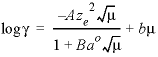, is multiplied times the equivalent fraction to obtain activity for the exchange species. In this equation,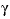is the activity coefficient,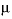is ionic strength (mol/L [mole per liter], assumed to be equal to mol/kgw [mole per kilogram water]), A and B are constants at a given temperature and pressure,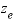is the number of equivalents of exchanger in the exchange species, and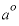and b are ion-specific parameters. Optionally, gamma or -g [ amma ].

Debye-Hückel a --Parameter ao in the WATEQ activity-coefficient equation.

Debye-Hückel b --Parameter b in the WATEQ activity-coefficient equation.

active_fraction_coefficient --Parameter for changing log_k as a function of the exchange sites occupied (Appelo, 1994a). The active-fraction model is useful for modeling sigmoidal exchange isotherms and proton exchange on organic matter (see http://www.hydrochemistry.eu/exmpls/a_f.html, accessed June 25, 2012).

Line 4: -davies

-davies --Indicates the Davies equation will be used to calculate an activity coefficient. If -gamma or -davies is not input for an exchange species, the activity of the species is equal to its equivalent fraction. If -davies is entered, then an activity coefficient of the form of the Davies equation,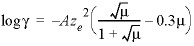, is multiplied times the equivalent fraction to obtain activity for the exchange species. In this equation,is the activity coefficient,is ionic strength, A is a constant at a given temperature, andis the number of equivalents of exchanger in the exchange species. Optionally, davies or -d [ avies ].

###### Notes

Lines 1 and 2 may be repeated as necessary to define all of the exchange reactions, with Line 1 preceding Line 2 for each exchange species. One identity reaction that defines the exchange master species (in the Example data block, Lines 1a and 2a, 1d and 2d) and one reference half-reaction are needed for each exchanger. The identity reaction has a log K of 0.0. The reference half-reaction for each exchanger also will have a log K of 0.0 (in the Example data block, Lines 1b and 2b, 1e and 2e); in the default database file the reference half-reaction is Na + + X - = NaX. Multiple exchangers may be defined simply by defining multiple exchange master species and additional half-reactions involving these master species, as in this Example data block.

Activities of exchange species may be expressed as equivalent or mole fractions of the species (Gaines-Thomas or Vanselow convention, respectively), or as fractions of the exchange sites occupied (Gapon convention). All three conventions can be used in PHREEQC (see http://www.hydrochemistry.eu/pub/ap_pa02.pdf, accessed June 25, 2012). In the databases, the Gaines-Thomas convention is used.

Cation exchange experiments with heterovalent exchange in which the salinity of the solutions is varied (for example, exchange of 2Na + for Ca2+ at varying Cl- concentrations) can be modeled better when exchange is calculated with molal concentrations for solute species instead of activities. This implies that the activity coefficients of solute cations and exchangeable species are the same, perhaps because a large part of cation exchange in soils and sediments takes part in the electrostatic double layer. Accordingly, PHREEQC permits the activity coefficient for exchangeable species to be defined in the same way as the solute species. The -gamma identifier allows the equivalent fraction to be multiplied by an activity coefficient by using the WATEQ Debye-Hückel equation. Similarly, when using the llnl.dat database, -llnl_gamma can be used to multiply the equivalent fraction by the activity coefficient that is defined according to the conventions of the llnl.dat database. The Davies equation can be used to calculate the activity coefficient of the exchange species by specifying the -davies identifier. The use of these equations is strictly empirical and is motivated by the observation that these activity corrections provide a better fit to some experimental data.

Temperature dependence of log K can be defined with the standard enthalpy of reaction ( -delta_h ) using the Van’t Hoff equation or with an analytical expression ( -analytical_expression ). Sometimes it is useful to offset a log K from zero for parameter fitting, or to account for dependencies among log K values, in which case the -add_log_k identifier can be used to add the value defined by a named analytical expression (MIX_EQUILIBRIUM_PHASES) to the log K of the exchange species. See SOLUTION_SPECIES for examples.

The identifier -no_check can be used to disable checking charge and elemental balances (see SOLUTION_SPECIES) and allows the Gapon exchange convention to be used (See http://www.hydrochemistry.eu/a&p/6/exch_phr.pdf, accessed June 25, 2012).

###### Example problems

The keyword EXCHANGE_SPECIES is used in example problems 12, 13, 18, and 21. See also the databases Amm.dat , iso.dat , llnl.dat , phreeqc.dat , pitzer.dat , and wateq4f.dat .

###### Related keywords

EXCHANGE, EXCHANGE_MASTER_SPECIES, SAVE exchange , SOLUTION_SPECIES, and USE exchange .

| Next || Previous || Top |